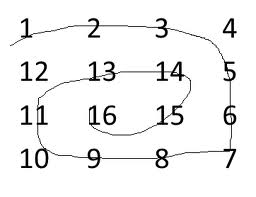# C++ Program to Print matrix in antispiral form

• Last Updated : 06 Jan, 2022

Given a 2D array, the task is to print matrix in anti spiral form:
Examples:Output: 16 15 14 13 12 11 10 9 8 7 6 5 4 3 2 1

```Input : arr[] = {1, 2, 3, 4
5, 6, 7, 8
9, 10, 11, 12
13, 14, 15, 16};
Output : 10 11 7 6 5 9 13 14 15 16 12 8 4 3 2 1

Input :arr[] = {1, 2, 3, 4, 5, 6
7, 8, 9, 10, 11, 12
13, 14, 15, 16, 17, 18};
Output : 11 10 9 8 7 13 14 15 16 17 18 12 6 5 4 3 2 1```

The idea is simple, we traverse matrix in spiral form and put all traversed elements in a stack. Finally one by one elements from stack and print them.

## C++

 `// C++ program to print matrix in anti-spiral form``#include ``using` `namespace` `std;``#define R 4``#define C 5`` ` `void` `antiSpiralTraversal(``int` `m, ``int` `n, ``int` `a[R][C])``{``    ``int` `i, k = 0, l = 0;`` ` `    ``/*  k - starting row index``        ``m - ending row index``        ``l - starting column index``        ``n - ending column index``        ``i - iterator  */``    ``stack<``int``> stk;`` ` `    ``while` `(k <= m && l <= n)``    ``{``        ``/* Print the first row from the remaining rows */``        ``for` `(i = l; i <= n; ++i)``            ``stk.push(a[k][i]);``        ``k++;`` ` `        ``/* Print the last column from the remaining columns */``        ``for` `(i = k; i <= m; ++i)``            ``stk.push(a[i][n]);``        ``n--;`` ` `        ``/* Print the last row from the remaining rows */``        ``if` `( k <= m)``        ``{``            ``for` `(i = n; i >= l; --i)``                ``stk.push(a[m][i]);``            ``m--;``        ``}`` ` `        ``/* Print the first column from the remaining columns */``        ``if` `(l <= n)``        ``{``            ``for` `(i = m; i >= k; --i)``                ``stk.push(a[i][l]);``            ``l++;``        ``}``    ``}`` ` `    ``while` `(!stk.empty())``    ``{``        ``cout << stk.top() << ``" "``;``        ``stk.pop();``    ``}``}`` ` `/* Driver program to test above functions */``int` `main()``{``    ``int` `mat[R][C] =``    ``{``        ``{1,  2,  3,  4,  5},``        ``{6,  7,  8,  9,  10},``        ``{11, 12, 13, 14, 15},``        ``{16, 17, 18, 19, 20}``    ``};`` ` `    ``antiSpiralTraversal(R-1, C-1, mat);`` ` `    ``return` `0;``}`

Output:

`12 13 14 9 8 7 6 11 16 17 18 19 20 15 10 5 4 3 2 1 `

Please refer complete article on Print matrix in antispiral form for more details!

My Personal Notes arrow_drop_up